# DAV Class 8 Maths Chapter 11 Brain Teasers Solutions

The DAV Maths Class 8 Solutions and DAV Class 8 Maths Chapter 11 Brain Teasers Solutions of Understanding Quadrilaterals offer comprehensive answers to textbook questions.

## DAV Class 8 Maths Ch 11 Brain Teasers Solutions

Question 1A.
Tick (✓) the correct option.

(i) The number of diagonals a pentagon has is
(a) 2
(b) 4
(c) 5
(d) 3
Solution:
A pentagon has three diagonals.
Thus, option (d) is the correct answer.

(ii) A parallelogram having its adjacent sides equal is a
(a) trapezium
(b) rhombus
(c) triangle
(d) rectangle
Solution:
A rhombus is a parallelogram in which adjacent sides are equal.
Thus, option (b) is the correct answer.

(iii) A seven-sided polygon is called a
(a) pentagon
(b) octagon
(c) hexagon
(d) heptagon
Solution:
A seven-sided polygon is called a heptagon.
Thus, option (d) is the correct answer.

(iv) Regular polygons are
(a) equiangular only
(b) equilateral only
(c) equiangular and equilateral
(d) None of the options
Solution:
Regular polygons are equiangular as well as equilateral.
Thus, option (c) is the correct answer.

(v) Which of the given polygons have equal diagonals?
(a) square
(b) rhombus
(c) parallelogram
(d) trapezium
Solution:
Gut of the given quadrilaterals, only the square has equal diagonals.
Thus, option (a) is the correct answer.Question 1B.

(i) Name two polygons whose diagonals bisect each other at right angles.
Solution:
Diagonals of rhombus and squares bisect each other at right angles.

(ii) In a parallelogram ABCD, if ∠B = 75°, find measure of ∠C.Solution:
Let ABCD be a parallelogram in which AD || BC
Given: ∠B = 75°
∠B + ∠C = 180° (Interior angles on the same side of the transversal)
⇒ ∠75° + ∠C = 180°
⇒ ∠C = 180° – 75° = 105°
⇒ ∠C = 105°

(iii) What is the measure of each exterior angle of a regular octagon?
Solution:
We know that the sum of the measures of the exterior angles of any polygon is irrespective of the number of sides it has.
Let each angle of a regular octagon be x.
∴ The measure of all the exterior angles of a regular octagon = 360°
⇒ 8x = 360°
⇒ x = 45°

(iv) Find the measure of x in the given figure.Solution:
Sum of the measures of exterior angles of given quadrilateral = 360°
⇒ 110° + 65° + 80° + x = 360°
⇒ 255° + x = 360°
⇒ x = 360° – 255°
⇒ x = 105°

(v) Find each angle of a regular pentagon.
Solution:
Number of sides of a regular pentagon, n = 5
Each angle of a regular pentagon = $$\frac{(n-2) \times 180^{\circ}}{n}$$
= $$\frac{(5-2) \times 180^{\circ}}{5}$$
= $$\frac{3 \times 180^{\circ}}{5}$$
= 108°Question 2.
ABCD is a parallelogram. $$\overline{\mathrm{AP}}$$ bisects ∠A and $$\overline{\mathrm{CQ}}$$ bisects ∠C. P lies on $$\overline{\mathrm{CD}}$$ and Q lies on $$\overline{\mathrm{AB}}$$. Show that:
(i) $$\overline{\mathrm{AP}} \| \overline{\mathrm{CQ}}$$
(ii) AQCP is a parallelogram.
Solution: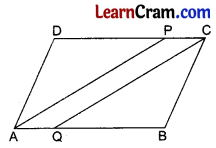∠A = ∠C [Opposite angles of a parallelogram]
∴ $$\frac{\angle \mathrm{A}}{2}=\frac{\angle \mathrm{C}}{2}$$
⇒ ∠DAP = ∠BCQ
AD = BC [Opposite Sides of a Parallelogram]
∠ADP = ∠CBQ [Opposite Angles of a Parallelogram]
∴ $$\overline{\mathrm{AP}}=\overline{\mathrm{CQ}}$$ [C.P.C.T.]
and $$\overline{\mathrm{DP}}=\overline{\mathrm{BQ}}$$ [C.P.C.T.]
$$\overline{\mathrm{DC}}=\overline{\mathrm{AB}}$$ [Opposite Sides of a Parallelogram]
∴ $$\overline{\mathrm{DC}}-\overline{\mathrm{DP}}=\overline{\mathrm{AB}}-\overline{\mathrm{BQ}}$$
$$\overline{\mathrm{PC}}=\overline{\mathrm{AQ}}$$
∴ $$\overline{\mathrm{PC}} \| \overline{\mathrm{AQ}}$$
∴ AQCP is a parallelogram. [Parallelogram prop.]
∴ $$\overline{\mathrm{AP}} \| \overline{\mathrm{CQ}}$$. [Opposite sides of a || gram]

Question 3.
In the given figure ABCD is a quadrilateral in which $$\overline{\mathrm{AB}}=\overline{\mathrm{AD}}$$ and $$\overline{\mathrm{BC}}=\overline{\mathrm{DC}}$$. Diagonals $$\overline{\mathrm{AC}}$$ and $$\overline{\mathrm{BD}}$$ intersect each other at O. Show that:
(ii) ΔAOB ≅ ΔAOD
(iii) $$\overline{\mathrm{AC}} \perp \overline{\mathrm{BD}}$$
(iv) $$\overline{\mathrm{AC}}$$ bisects $$\overline{\mathrm{BD}}$$
Solution:$$\overline{\mathrm{AB}}=\overline{\mathrm{AD}}$$ [Given]
$$\overline{\mathrm{BC}}=\overline{\mathrm{DC}}$$ [Given]
$$\overline{\mathrm{AC}}=\overline{\mathrm{AC}}$$ [Common]
∴ ∠1 = ∠2 [C.P.C.T.]

(ii) In ΔAOB and ΔAOD
$$\overline{\mathrm{AB}}=\overline{\mathrm{AD}}$$ [Given]
∠1 = ∠2 [From I]
$$\overline{\mathrm{AO}}=\overline{\mathrm{AO}}$$ [Common]
∴ ΔAOB ≅ ΔAOD [SAS]

(iii) ∠AOB = ∠AOD [C.P.C.T.]
But ∠AOB + ∠AOD = 180° [Linear pairs]
∴ ∠AOB = ∠AOD = 90°
∴ $$\overline{\mathrm{AC}} \perp \overline{\mathrm{BD}}$$

(iv) BO = OD [C.P.C.T.]
Hence $$\overline{\mathrm{AC}}$$ bisects $$\overline{\mathrm{BD}}$$.Question 4.
ABCD is a quadrilateral in which all four sides are equal. Show that both pairs of opposite sides are parallel.
Solution: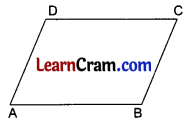$$\overline{\mathrm{AB}}=\overline{\mathrm{BC}}=\overline{\mathrm{CD}}=\overline{\mathrm{DA}}$$
∴ ABCD is a rhombus. [Rhombus property]
So, $$\overline{\mathrm{AB}} \| \overline{\mathrm{DC}}$$ and $$\overline{\mathrm{AD}} \| \overline{\mathrm{BC}}$$ [Rhombus property]

Question 5.
In a quadrilateral ABCD, ∠A + ∠D = 180°. Does this mean AB || DC? Why? What special name does this quadrilateral have?
Solution: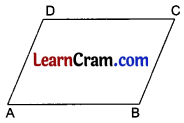In ABCD,
∠A + ∠D = 180° [Given]
∴ AB || DC

### DAV Class 8 Maths Chapter 11 HOTS

Question 1.
Prove that the interior angle of a regular pentagon is three times the exterior angle of a regular decagon.
Solution:
Each interior angle of a regular pentagon = $$\frac{(5-2) \times 180^{\circ}}{5}$$
= $$\frac{3 \times 180^{\circ}}{5}$$
= 108°
The sum of exterior angles of a regular decagon = 360°
∴ The measure of each exterior angle of a regular decagon = $$\frac{360^{\circ}}{10}$$ = 36°
Clearly, the interior angle of a regular pentagon = 108°
= 3 × 36°
= 3 × exterior angle of a regular decagon
Hence proved.Question 2.
In the given figure, ABCDE is a regular pentagon. If ∠EBD = 36°, find ∠1, ∠2 and ∠3.Solution:
Each interior angle of a regular pentagon = $$\frac{(5-2) \times 180^{\circ}}{5}$$ = 108°
So, ∠1 = 108°
∆ABE is an isosceles triangle in which AB = AE
∴ ∠ABE = ∠AEB = x (say)
Then, ∠A + ∠ABE + ∠AEB = 180° (Angle Sum Property)
⇒ ∠1 + x + x = 180°
⇒ 108° + 2x = 180°
⇒ 2x = 180° – 108°
⇒ 2x = 72°
⇒ x = 36°
∴ ∠2 = 36° and ∠ABE = 36°
Also, the measure of ∠B = 108° (Interior angle of a regular pentagon)
⇒ ∠ABE + ∠EBD + ∠DBC = 108°
⇒ 36° + 36° + ∠3 = 108°
⇒ ∠3 + 72° = 108°
⇒ ∠3 = 108° – 72° = 36°
Thus, ∠1 = 108°, ∠2 = 36°, ∠3 = 36°

### DAV Class 8 Maths Chapter 11 Enrichment Questions

Question 1.
How many sides have a regular polygon, each angle of which is of measure
(i) 156°
(ii) 108°
Solution:
(i) Let the number of sides of a regular polygon be n.
Each angle of a regular polygon = 156° (Given)
⇒ $$\frac{(n-2) \times 180^{\circ}}{n}$$ = 156°
⇒ (n – 2) × 180° = 156° × n
⇒ 180° n – 360° = 156° n
⇒ 180° n – 156° n = 360°
⇒ 24° n = 360°
⇒ n = 15
Thus, the number of sides of the regular polygon = 15

(ii) Let the number of sides of a regular polygon be n.
Each angle of a regular polygon = 108° (Given)
⇒ $$\frac{(n-2) \times 180^{\circ}}{n}$$ = 108°
⇒ 180° n – 360° = 108° n
⇒ 180° n – 108° n = 360°
⇒ 72° n = 360°
⇒ n = 5
Thus, the number of sides of the regular polygon = 5Question 2.
In the given figure, ABCD is a trapezium. If x = $$\frac{4}{3}$$y, y = $$\frac{3}{8}$$z, then find the value of x.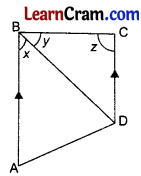Solution:
In the given trapezium, AB || DC and BC is a transversal.
Since interior angles on the same side of the transversal are supplementary.
∴ (x + y) + z = 180°
⇒ $$\frac{4}{3} y+y+\frac{8 y}{3}$$ = 180°
(∵ x = $$\frac{4}{3}$$y (given) and y = $$\frac{3}{8}$$z (given) ⇒ z = $$\frac{8y}{3}$$)
⇒ $$\frac{4 y+3 y+8 y}{3}$$ = 180°
⇒ $$\frac{15y}{3}$$ = 180°
⇒ y = 36°
Using the value of y, we get
x = $$\frac{4}{3}$$y
⇒ x = $$\frac{4}{3}$$ × 36°
⇒ x = 48°

Question 1.
Prove that the sum of all exterior angles of a quadrilateral is 360°.
Solution:Let a, b, c, and d be the exterior angles of an ABCD.
∠1 + ∠a = 180° [Linear pairs]
∠2 + ∠b = 180° [Linear pairs]
∠3 + ∠c = 180° [Linear pairs]
∠4 + ∠d = 180° [Linear pairs]
Adding ∠1 + ∠2 + ∠3 + ∠4 + ∠a + ∠b + ∠c + ∠d = 720°
⇒ 360° + ∠a + ∠b + ∠c + ∠d = 720° [Sum of all interior angles of quadrilateral is 360°]
⇒ ∠a + ∠b + ∠c + ∠d = 720° – 360°
⇒ ∠a + ∠b + ∠c + ∠d = 360°

Question 2.
The two adjacent sides of a parallelogram are in the ratio 5 : 6, find the sides of the parallelogram if its perimeter is 308 cm.
Solution:In the given figure,
Let AD = 5x cm and AB = 6x cm
∴ Perimeter of the parallelogram ABCD = 2[AB + AD]
∴ 2[6x + 5x] = 308
⇒ 2 × 11x = 308
⇒ 22x = 308
⇒ x = 14
Hence AB = 6 × 14 = 84 cm and AD = 5 × 14 = 70 cm.Question 3.
The diagonals of a rhombus are in the ratio 3 : 4. If its each side is 10 cm, find the lengths of its diagonal.
Solution: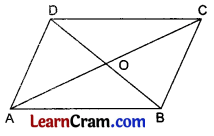Let BD = 3x cm and AC = 4x cm
∴ OA = 2x cm and OB = $$\frac{3}{2}$$x cm
[∵ Diagonals of a rhombus bisect each other at 90°]
In rt. ΔAOB
(OA)2 + (OB)2 = (AB)2 [By Pythagoras Theorem]
⇒ (2x)2 + ($$\frac{3}{2}$$x)2 = (10)2
⇒ 4x2 + $$\frac{9}{4}$$x2 = 100
⇒ $$\frac{25}{4}$$x2 = 100
⇒ x2 = $$\frac{100 \times 4}{25}$$
⇒ x2 = 16
⇒ x = 4
Hence AC = 4 × 6 = 24 cm and BD = 4 × 5 = 20 cm.

Question 4.
The difference between two adjacent angles of a parallelogram is 30°, find the angles.
Solution: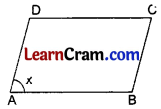Let ∠A = x°
∠A + ∠B = 180° [Sum of adjacent angles of a ||gm]
⇒ x° + ∠B = 180°
∴ ∠B = 180° – x°
But ∠A – ∠B = 30° [Given]
⇒ x° – (180° – x°) = 30°
⇒ x° – 180° + x° = 30°
⇒ 2x° = 30° + 180°
⇒ 2x° = 210°
⇒ x° = 105°
Hence ∠A = 105° and ∠B = 180° – 105° = 75°.Question 5.
In the given figure, AB || DC and AD = BC. If AD || CE and ∠A = 70°, find all the angles of the trapezium ABCD.
Solution: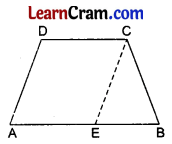AE || DC
∴ AECD is a parallelogram
∠A = 70° [Given]
∴ ∠CEB = 70° [Corresponding angles]
But CE = AD = BC [Given]
∴ ∠CEB = ∠B = 70° [Angles opposite to equal sides in a triangle]
∠A + ∠D = 180° [Adjacent angles]
⇒ 70° + ∠D = 180°
⇒ ∠D = 180° – 70° = 110°
∠B + ∠C = 180° [Adjacent angles]
70° + ∠C = 180°
∴ ∠C = 180° – 70° = 110°

Question 6.
Mid-points of opposite sides of a parallelogram are joined as shown in the figure.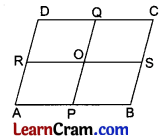Show that:
(i) APQD is a parallelogram
(ii) $$\overline{\mathrm{AD}} \| \overline{\mathrm{PQ}}$$
(iii) ABSR is a parallelogram
(iv) APOR is a parallelogram.
Solution:
(i) $$\overline{\mathrm{AB}}=\overline{\mathrm{DC}}$$ [Opposite Sides of a Parallelogram]
$$\overline{\mathrm{AB}} \| \overline{\mathrm{DC}}$$ [Opposite Sides of a Parallelogram]
$$\frac{1}{2} \overline{\mathrm{AB}}$$ || and = $$\frac{1}{2} \overline{\mathrm{AB}}$$
∴ AP || and = DQ
∴ APQD is a parallelogram
(If a pair of opposite sides of a quadrilateral are equal and parallel, then it is a parallelogram)
(ii) $$\overline{\mathrm{AD}} \| \overline{\mathrm{PQ}}$$ [Opposite Sides of a Parallelogram]
(iii) $$\overline{\mathrm{AD}}$$ = and || $$\overline{\mathrm{BC}}$$ [Opposite Sides of a Parallelogram]
∴ $$\frac{1}{2} \overline{\mathrm{AD}}$$ = and || $$\frac{1}{2} \overline{\mathrm{BC}}$$
$$\overline{\mathrm{AR}}$$ = and || $$\overline{\mathrm{BS}}$$
∴ ABSR is a Parallelogram.
(iv) O is the midpoint of $$\overline{\mathrm{PQ}}$$ and $$\overline{\mathrm{RS}}$$
[The line joining the mid-points of opposite sides of a parallelogram bisect each other]
∴ $$\overline{\mathrm{AP}}$$ = and || $$\overline{\mathrm{RO}}$$
and $$\overline{\mathrm{AR}}$$ = and || $$\overline{\mathrm{PO}}$$
Hence APOR is a parallelogram.Question 7.
Diagonal AC of a parallelogram ABCD bisects ∠A.
(i) Does AC bisect ∠C also? Give reason.
(ii) Is ABCD a rhombus? Give reason.Solution:
∠1 = ∠2 [Given]
∠1 = ∠3
and ∠2 = ∠4 [Alternate Angles]
∴ ∠3 = ∠4
So, AC also bisects ∠C
(ii) In parallelogram ABCD, diagonal AC, bisects ∠A and ∠C both.
∴ ABCD is a Rhombus [Rhombus Property]

Question 8.
ABCD is a parallelogram in which the diagonals bisect each other at right angles. Show that it is a rhombus.
Solution:In ∆AOB and ∆BOC
OA = OC [Given]
∠AOB = ∠COB = 90° [Given]
OB = OB [Common]
∴ ∆AOB ≅ ∆COB [SAS]
∴ AB = BC [C.P.C.T.]
Similarly BC = CD = DA
Hence ABCD is a Rhombus.Question 9.
ABCD is a quadrilateral in which the diagonals bisect each other. Show that it is a parallelogram.
Solution: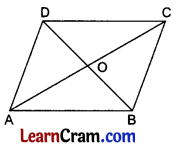In ∆AOB and ∆COD,
OA = OC
OB = OD [Given]
∠AOB = ∠COD [Vertically Opposite Angles]
∴ ∆AOB ≅ ∆COD (SAS)
∴ AB = DC [C.P.C.T.]
and ∠OAB = ∠OCD [C.P.C.T.]
But these are alternate angles.
∴ AB || DC.
Hence ABCD is a Parallelogram.